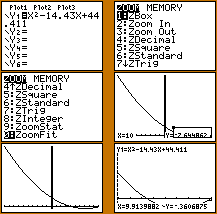Go to contentHow to solve a quadratic equation on a GDC (graphical display calculator). This explanation uses the TI-82/83/84 calculators.

1. Press Y= to select the equation entry menu.
3. You could press press GRAPH but it is better to press ZOOM then press 6 or select ZStandard.
4. You will see a display of your graph. Press ZOOM again then press 1 or select ZBox
5. Once back on the graph display, use the cursor keys and press ENTER at the top left and bottom right to create a zoom box

By repeated zooming in and use of the TRACE function you can obtain an accurate estimate of where the curve crosses the x-axis.You can get a better display of the maths by downloading special TeX fonts from jsMath. In the meantime, we will do the best we can with the fonts you have, but it may not be pretty and some equations may not be rendered correctly.

## Glossary

### equation

A statement that two mathematical expressions are equal.

### function

A rule that connects one value in one set with one and only one value in another set.

### gdc

graphic display calculator

### graph

A diagram showing a relationship between two variables.
The diagram shows a vertical y axis and a horizontal x axis.

A mathematical expression of the 2nd degree; having a x² term but no higher one.

### solve

To find the answer or solution to a problem.

### union

The union of two sets A and B is the set containing all the elements of A and B.

Full Glossary List

## This question appears in the following syllabi:

SyllabusModuleSectionTopicExam Year
AP Calculus AB (USA)1Algebra and FunctionsQuadratic solving-
AP Calculus BC (USA)1Algebra and FunctionsQuadratic solving-
AQA A-Level (UK - Pre-2017)C1Algebra and FunctionsQuadratic solving-
AQA AS Maths 2017Pure MathsAlgebraSolving with Discriminants-
AQA AS/A2 Maths 2017Pure MathsAlgebraSolving with Discriminants-
CBSE X (India)AlgebraPolynomialsZeros-
CCEA A-Level (NI)C1Algebra and FunctionsQuadratic solving-
CIE A-Level (UK)P1Algebra and FunctionsQuadratic solving-
Edexcel A-Level (UK - Pre-2017)C1Algebra and FunctionsQuadratic solving-
Edexcel AS Maths 2017Pure MathsQuadraticsSolving with Discriminants-
Edexcel AS/A2 Maths 2017Pure MathsQuadraticsSolving with Discriminants-
I.B. Higher Level2Algebra and FunctionsQuadratic solving-
I.B. Standard Level2Algebra and FunctionsQuadratic solving-